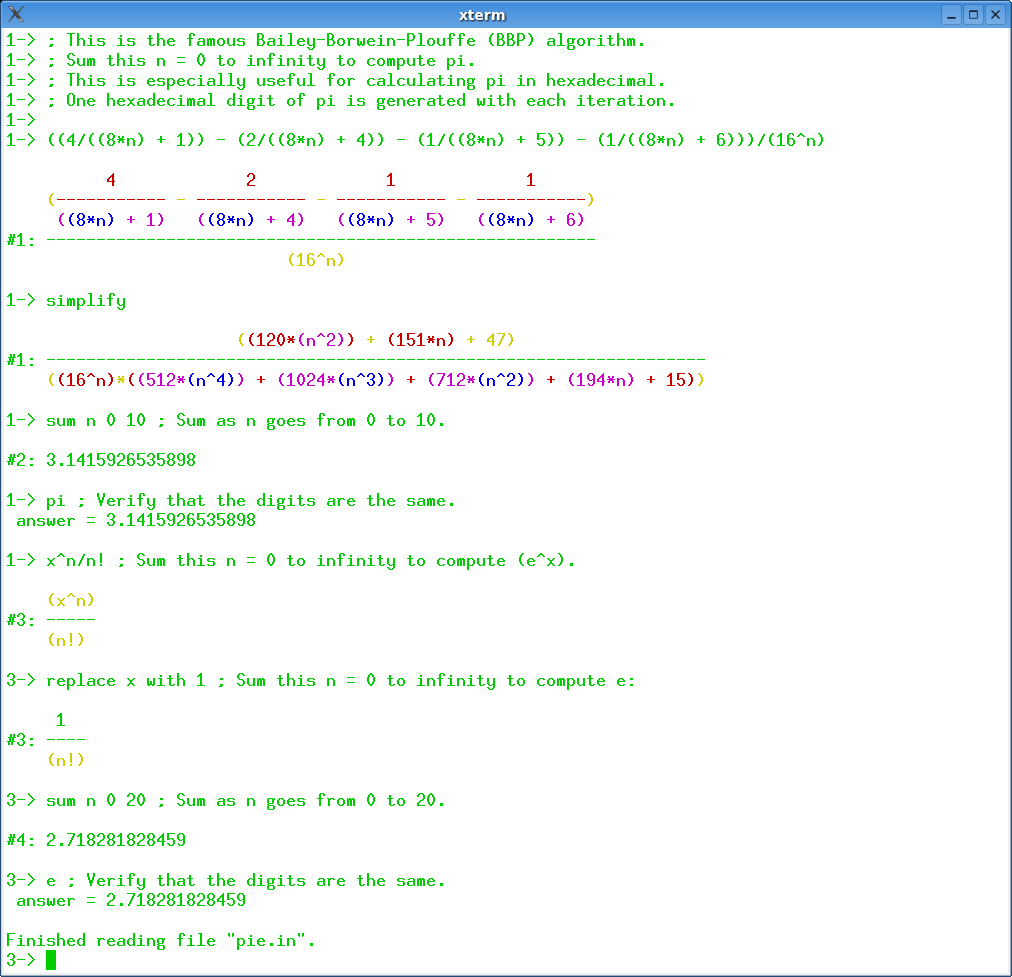## 8 Search Results

### Fermat

Fermat is a super calculator - computer algebra system, in which the basic items being computed can be rational numbers, modular numbers, elements of finite fields, multivariable polynomials, multivariable rational functions, or multivariable polynomials modulo other polynomials. Fermat is available for Mac OS, Windows, Unix, and Linux. It is shareware. The basic “ground ring" F is the field of rational numbers. One may choose to work modulo a specified integer n, thereby changing the ground ring F from Q to Z/n. On top of this may be attached any number of unevaluated variables t_1, t_2, .. t_n., thereby creating the polynomial ring F[t_1, t_2, .. t_n] and its quotient field, the rational functions. Further, polynomials p, q, .. can be chosen to mod out with, creating the quotient ring F(t_1, t_2, ..)/[p, q, ...]. It is possible to allow Laurent polynomials. Once the computational ring is established in this way, all computations are of elements of this ring.

### LAPACK

LAPACK is written in Fortran77 and provides routines for solving systems of simultaneous linear equations, least-squares solutions of linear systems of equations, eigenvalue problems, and singular value problems. The associated matrix factorizations (LU, Cholesky, QR, SVD, Schur, generalized Schur) are also provided, as are related computations such as reordering of the Schur factorizations and estimating condition numbers. Dense and banded matrices are handled, but not general sparse matrices. In all areas, similar functionality is provided for real and complex matrices, in both single and double precision.

### LiE

LiE is the name of a software package that enables mathematicians and physicists to perform computations of a Lie group theoretic nature. It focuses on the representation theory of complex semisimple (reductive) Lie groups and algebras, and on the structure of their Weyl groups and root systems. LiE does not compute directly with elements of the Lie groups and algebras themselves; it rather computes with weights, roots, characters and similar objects.

### Mathomatic

Mathomatic is a free, portable, general-purpose CAS (Computer Algebra System) and calculator software that can symbolically solve, simplify, combine, and compare equations, perform complex number and polynomial arithmetic, etc. It does some calculus and is very easy to use.

More information### Matlab

MATLAB is a high-level language and interactive environment that enables you to perform computationally intensive tasks faster than with traditional programming languages such as C, C++, and Fortran.

### PolyBoRi

The core of PolyBoRi is a C++ library, which provides high-level data types for Boolean polynomials and monomials, exponent vectors, as well as for the underlying polynomial rings and subsets of the powerset of the Boolean variables. As a unique approach, binary decision diagrams are used as internal storage type for polynomial structures. On top of this C++-library we provide a Python interface. This allows parsing of complex polynomial systems, as well as sophisticated and extendable strategies for Gröbner base computation. PolyBoRi features a powerful reference implementation for Gröbner basis computation.

More information### Scilab Image Processing Toolbox

SIP provides image processing, pattern recognition, and computer vision routines for SciLab, a Matlab-like matrix-oriented programming environment. SIP is able to read/write images in almost 90 major formats, including JPEG, PNG, BMP, GIF, FITS, and TIFF. It includes routines for filtering, segmentation, edge detection, morphology, curvature, fractal dimension, distance transforms, multiscale skeletons, and more.